login  home  contents  what's new  discussion  bug reports help  links  subscribe  changes  refresh  edit

In practice we frequently need to deal with composite functions. This is frequently formulated as y depends in x where x is a function (say of argument t). Unlike some other systems in FriCAS one have to be explicit with dependencies. This make simple cases slightly more verbose, but allows clean handling of more complicated cases. For concretness consider the following situation: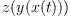where z is simple function of y, x is simple function of t say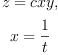and y is arbitrary. We want to compute second derivative of our function.

In FriCAS we can express is in very natural way. First define y as an operator to represent arbitrary function:

fricas
(1) -> y := operator 'y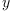(1)
Type: BasicOperator?

Now we just translate math to FriCAS syntax:

fricas
x := 1/t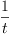(2)
Type: Fraction(Polynomial(Integer))
fricas
z := c*x*y(x)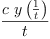(3)
Type: Expression(Integer)
fricas
D(z, t, 2)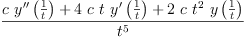(4)
Type: Expression(Integer)

 Subject:   Be Bold !! ( 14 subscribers )